# Closure properties of Regular languages

Closure properties on regular languages are defined as certain operations on regular language which are guaranteed to produce regular language. Closure refers to some operation on a language, resulting in a new language that is of same “type” as originally operated on i.e., regular.

Regular languages are closed under following operations.

Consider L and M are regular languages:

Kleen Closure:
RS is a regular expression whose language is L, M. R* is a regular expression whose language is L*.

1.      Positive closure:
RS is a regular expression whose language is L, M.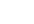is a regular expression whose language is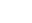.

2.      Complement:
The complement of a language L (with respect to an alphabet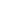such that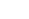contains L) is–L. Sinceis surely regular, the complement of a regular language is always regular.

3.      Reverse Operator:
Given language L,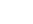is the set of strings whose reversal is in L.
Example: L = {0, 01, 100};={0, 10, 001}.
Proof: Let E be a regular expression for L. We show how to reverse E, to provide a regular expression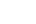for.

4.      Complement:
The complement of a language L (with respect to an alphabetsuch thatcontains L) is–L. Sinceis surely regular, the complement of a regular language is always regular.

5.      Union:
Let L and M be the languages of regular expressions R and S, respectively.Then R+S is a regular expression whose language is(L U M).

6.      Intersection:
Let L and M be the languages of regular expressions R and S, respectively then it a regular expression whose language is L intersection M.
proof: Let A and B be DFA’s whose languages are L and M, respectively. Construct C, the product automaton of A and B make the final states of C be the pairs consisting of final states of both A and B.

7.      Set Difference operator:
If L and M are regular languages, then so is L – M = strings in L but not M.

Proof: Let A and B be DFA’s whose languages are L and M, respectively. Construct C, the product automaton of A and B make the final states of C be the pairs, where A-state is final but B-state is not.

8.      Homomorphism:
A homomorphism on an alphabet is a function that gives a string for each symbol in that alphabet. Example: h(0) = ab; h(1) =. Extend to strings by h(a1…an) =h(a1)…h(an). Example: h(01010) = ababab.

If L is a regular language, and h is a homomorphism on its alphabet, then h(L)= {h(w) | w is in L} is also a regular language.
Proof: Let E be a regular expression for L. Apply h to each symbol in E. Language of resulting R, E is h(L).

9.      Inverse Homomorphism : Let h be a homomorphism and L a language whose alphabet is the output language of h.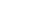(L) = {w | h(w) is in L}.

Note: There are few more properties like symmetric difference operator, prefix operator, substitution which are closed under closure properties of regular language.

Decision Properties:
Approximately all the properties are decidable in case of finite automaton.

(i) Emptiness

(ii) Non-emptiness

(iii) Finiteness

(iv) Infiniteness

(v) Membership

(vi) Equality

These are explained as following below.

(i) Emptiness and Non-emptiness:

·   Step-1: select the state that cannot be reached from the initial states & delete them (remove unreachable states).

·   Step 2: if the resulting machine contains at least one final states, so then the finite automata accepts the non-empty language.

·   Step 3: if the resulting machine is free from final state, then finite automata accepts empty language.

(ii) Finiteness and Infiniteness:

·         Step-1: select the state that cannot be reached from the initial state & delete them (remove unreachable states).

·         Step-2: select the state from which we cannot reach the final state & delete them (remove dead states).

·         Step-3: if the resulting machine contains loops or cycles then the finite automata accepts infinite language.

·         Step-4: if the resulting machine do not contain loops or cycles then the finite automata accepts infinite language.

(iii) Membership:
Membership is a property to verify an arbitrary string is accepted by a finite automaton or not i.e. it is a member of the language or not.

Let M is a finite automata that accepts some strings over an alphabet, and let ‘w’ be any string defined over the alphabet, if there exist a transition path in M, which starts at initial state & ends in anyone of the final state, then string ‘w’ is a member of M, otherwise ‘w’ is not a member of M.

(iv) Equality:
Two finite state automata M1 & M2 is said to be equal if and only if, they accept the same language. Minimise the finite state automata and the minimal DFA will be unique.